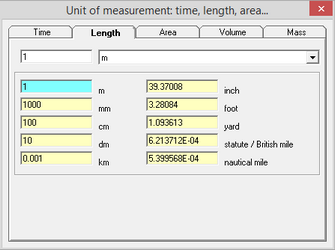﻿ Help > Unit converter (Part 2) > Length

# Length

Navigation:  Help > Unit converter (Part 2) >

# LengthTab "Length" of the dialog window "Unit converter (part 2)"

 Metre (m) The metre (Commonwealth spelling) or meter (American spelling) is the base unit of length in the International System of Units (SI). The metre is defined as the length of the path travelled by light in a vacuum in 1/299792458 of a second. The metre was originally defined in 1793 as one ten-millionth of the distance from the equator to the North Pole along a great circle, so the Earth's circumference is approximately 40000 km. In 1799, the metre was redefined in terms of a prototype metre bar (the actual bar used was changed in 1889). In 1960, the metre was redefined in terms of a certain number of wavelengths of a certain emission line of krypton-86. In 1983, the current definition was adopted. Millimetre (mm) 1 mm = 1/1000 m Centimetre (cm) A centimetre (international spelling as used by the International Bureau of Weights and Measures) or centimeter (American spelling) is a unit of length in the metric system, equal to one hundredth of a metre, centi being the SI prefix for a factor of 1/100.The centimetre was the base unit of length in the now deprecated centimetre–gram–second (CGS) system. Decimetre (dm) 1 dm = 1/10 m Kilometre (km) 1 km = 1000 m Inch (in) The inch (abbreviation: in or ″) is a unit of length in the (British) imperial and United States customary systems of measurement. It is equal to ​1⁄36 yard or ​1⁄12 of a foot. Derived from the Roman uncia ("twelfth"), the word inch is also sometimes used to translate similar units in other measurement systems, usually understood as deriving from the width of the human thumb. Foot (ft) The foot is  one of the Imperial units and U.S. customary units. The shortest way of writing the unit "foot" is by the abbreviation "ft" (or "ft."), or by a prime symbol ( ' ). One foot contains 12 inches. This is equal to 30.48 centimetres. It is called a foot, because it was originally based on the length of a foot. Yard (yd) The yard is an English unit of length, in both the British imperial and US customary systems of measurement, that comprises 3 feet or 36 inches. 1,760 yards is equal to 1 mile. Since 1959 it is by international agreement standardized as exactly 0.9144 meters. (The US survey yard is very slightly longer). Mile (mi) The mile is an English unit of length of linear measure equal to 5,280 feet, or 1,760 yards, and standardised as exactly 1,609.344 metres by international agreement in 1959. Geographic mile (or German mile) = 7.4204 km; Old Russian mile = 7.46760 km; Old Roman mile = 1.481 km. Nautical mile (nm) A nautical mile is a unit of measurement used in both air and marine navigation, and for the definition of territorial waters. Historically, it was defined as one minute (1/60 of a degree) of latitude along any line of longitude. Today the international nautical mile is defined as exactly 1852 metres (about 1.15 miles). The derived unit of speed is the knot, one nautical mile per hour.